# Chapter 10 Self Test

## Moving Bed Reactors

The elementary irreversible gas phase catalytic reactionis to be carried out in a moving bed reactor at constant temperature. The reactor contains 5 kg. of catalyst. The feed is stoichiometric in A and B. The entering concentration of A is 0.2 mol/dm3. The catalyst decay law is zero order with kD = 0.2 s–1. What catalyst loading rate is necessary to achieve 40% conversion?

k = 1.0 dm6/(mol•kg. cat•s)

vo = 1 dm3/s

Hint 1 What are the mole balance and the rate law?

Hint 2 What is the equation for catalyst activity as a function of loading rate?

Hint 3 What is the differential equation that combines the mole balance, activity law and rate law?

Hint 4 What is the solution to the equation in Hint 3?

Hint 5 What conversion will be achieved for a catalyst feed rate of 0.5 kg/s?

Hint 6 Sketch the catalyst activity as a function of catalyst weight (i.e., distance) down the length such for a catalyst feed rate of 0.5 kg/s.

Hint 7 What is the maximum conversion that could be achieved (i.e. at infinite catalyst loading rate)?

Hint 8 At what catalyst loading rate (kg/s) will the catalyst activity be exactly zero at the exit of the reactor?

Hint 1. Mole balance and rate laws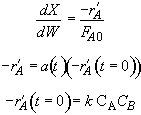Back to Hints

Back to Problem

Hint 2. Equation for catalyst activity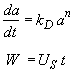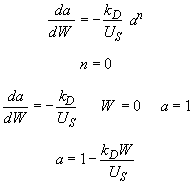Back to Hints

Back to Problem

Hint 3. Combine mole balance, rate law, decay law, and stoichiometry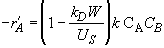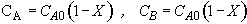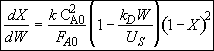(1)

Back to Hints

Back to Problem

Hint 4. Solution to Hint 3.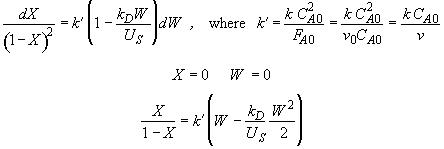Back to Hints

Back to Problem

Hint 5. What is conversion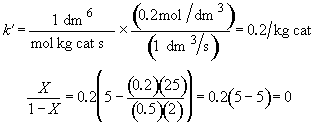Zero conversion. NONSENSE!

Back to Hints

Back to Problem

Hint 6. Sketch a vs. W

Look at a vs. W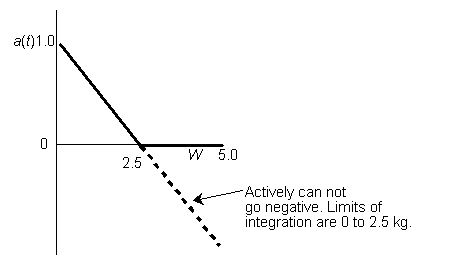Therefore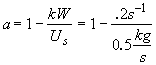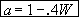(2)

when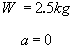therefore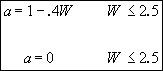(3)

Rearranging Equation (1) and integratingBack to Hints

Back to Problem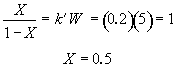Back to Hints

Back to Problem

Hint 9. Loading rate for which a is exactly zero at the exit of reaction.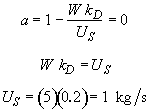at a loading of 1 kg/s the catalyst activity will be exactly zero at the exit of the reactor.

What does an activity of zero mean? Can catalyst activity be less than zero?

Back to Chapter 10